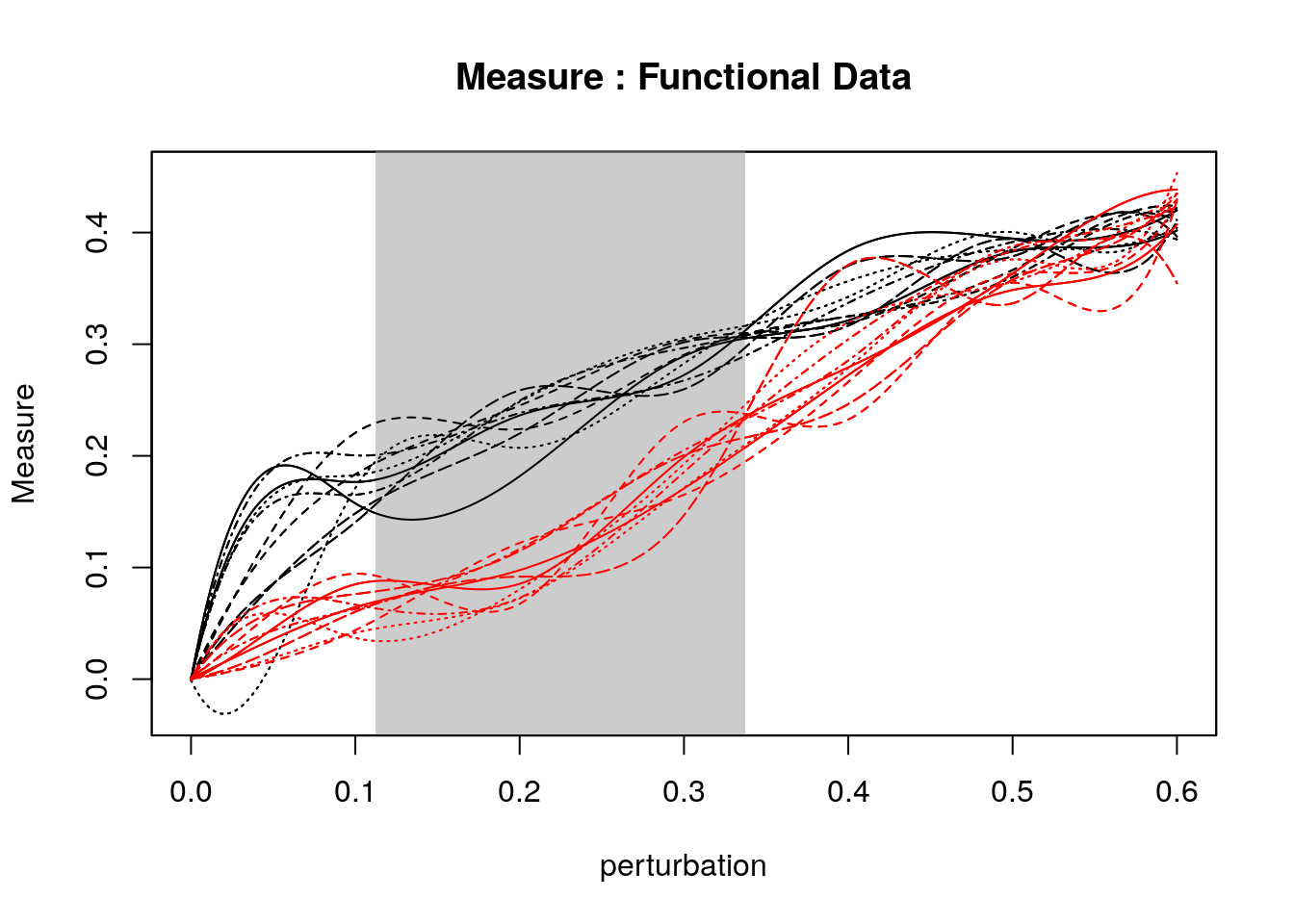# ROBIN

In network analysis, many community detection algorithms have been developed. However,their applications leave unaddressed one important question: the statistical validation of the results.

ROBIN (ROBustness In Network) is an R package for the validation of community detection it has a double aim: it studies the robustness of a community detection algorithm and compares the robustness of different community detection algorithms on the same network.

It provides: 1)A procedure to examine the robustness of a community detection algorithm against random perturbations of the original graph 2)Two tests to determine the statistical difference between stability measure curves 3)A routine to choose among different community detection algorithms the one that better fits the network of interest 4)A graphical interactive representation

``library("robin")``

# Preparation of the Graph

As input, the ROBIN package expects a network that can be read from different format: edgelist, pajek, graphml, gml, ncol, lgl, dimacs, graphdb and igraph graphs.

prepGraph function creates an igraph object from the input file. This step is necessary for ROBIN execution

``````my_network <- system.file("example/football.gml", package="robin")
graph <- prepGraph(file=my_network, file.format="gml")
graph``````
``````## IGRAPH 8e8827b U--- 115 613 --
## + attr: id (v/n), label (v/c), value (v/n)
## + edges from 8e8827b:
##   1--  2 1--  5 1-- 10 1-- 17 1-- 24 1-- 34 1-- 36 1-- 42 1-- 66 1-- 91
##  1-- 94 1--105 2-- 26 2-- 28 2-- 34 2-- 38 2-- 46 2-- 58 2-- 90 2--102
##  2--104 2--106 2--110 3--  4 3--  7 3-- 14 3-- 15 3-- 16 3-- 48 3-- 61
##  3-- 65 3-- 73 3-- 75 3--101 3--107 4--  6 4-- 12 4-- 27 4-- 41 4-- 53
##  4-- 59 4-- 73 4-- 75 4-- 82 4-- 85 4--103 5--  6 5-- 10 5-- 17 5-- 24
##  5-- 29 5-- 42 5-- 70 5-- 94 5--105 5--109 6-- 11 6-- 12 6-- 53 6-- 75
##  6-- 82 6-- 85 6-- 91 6-- 98 6-- 99 6--108 7--  8 7-- 33 7-- 40 7-- 48
##  7-- 56 7-- 59 7-- 61 7-- 65 7-- 86 7--101 7--107 8--  9 8-- 22 8-- 23
## + ... omitted several edges``````

# Random Graph

random creates the null model for the robustness procedure robinRobust. The graph argument must be the same returned by prepGraph function.

``````graphRandom <- random(graph=graph)
graphRandom``````
``````## IGRAPH 397edd2 U--- 115 613 --
## + attr: id (v/n), label (v/c), value (v/n)
## + edges from 397edd2:
##     1--  2   1-- 58   1-- 80   1-- 59  25-- 93  34-- 73  92--114
##    26-- 42   1-- 88   1--101   1-- 79  72-- 75   2-- 92   2-- 14
##    2-- 71  38--102   2-- 46   2-- 82   2-- 45  54-- 69   2-- 36
##  106--115  60-- 94   3--  4   3--  7  14-- 80   3-- 19   3-- 74
##    3-- 48   3--112   3-- 95  12-- 82  59-- 88   3--102  47--111
##    4-- 76  36-- 80   4-- 27  28--105  44-- 79  16--104  55-- 76
##    4-- 37   4-- 17   4-- 26  54--106   5-- 78   5-- 26  18-- 74
##    5-- 20   4-- 65   5-- 76   5-- 75   5-- 16   5-- 32   5-- 50
## + ... omitted several edges``````

# Plot graph

plotGraph function implements a graphical representation of the network with aid of networkD3 package.

``plotGraph(graph)``

# Create Community

To dectect communities using all the algorithms implemented in igraph package.

``methodCommunity(graph=graph, method="fastGreedy") #as community ``
``````## IGRAPH clustering fast greedy, groups: 6, mod: 0.55
## + groups:
##   \$`1`
##       7  14  16  33  40  48  61  65 101 107
##
##   \$`2`
##       8   9  10  17  22  23  24  42  47  50  52  54  68  69  74  78  79
##     89 105 109 111 112 115
##
##   \$`3`
##       1   2  20  26  30  31  34  36  38  46  56  80  81  83  90  94  95
##    102 104 106 110
##   + ... omitted several groups/vertices``````
``membershipCommunities(graph=graph, method="fastGreedy") #as membership``
``````##    3 3 5 5 5 5 1 2 2 2 5 5 4 1 4 1 2 6 4 3 6 2 2 2 5 3 4 6 5 3 3 4 1 3 4
##   3 6 3 4 1 5 2 6 4 6 3 2 1 6 2 5 2 5 2 4 3 6 6 6 6 1 4 6 6 1 6 6 2 2 5
##   6 4 5 2 5 6 6 2 2 3 3 5 3 5 5 4 6 6 2 3 5 6 6 3 3 6 6 6 5 4 1 3 5 3 2
##  3 1 5 2 3 2 2 6 6 2``````

# Community Plot

plotComm produces an interactive 3D plot of the communites detected by chosen algorithm.

``````members <- membershipCommunities(graph=graph, method="fastGreedy")
plotComm(graph=graph, members=members)``````

# Procedure to validate the robustness

robinRobust implements the validation of community robustness. In this example we use “vi” distance as stability measure, indipendent type procedure and louvain as community detection algorithm. Users can choose different measures (“nmi”,“split.join”, “adjusted.rand”) and algorithms (walktrap“,”edgeBetweenness“,”fastGreedy“,”spinglass“,”leadingEigen“,”labelProp“,”infomap“,”optimal“,”other").

``````proc <- robinRobust(graph=graph, graphRandom=graphRandom, measure="vi",
method="louvain", type="independent")``````
``````##  31
##  61
##  92
##  123
##  153
##  184
##  215
##  245
##  276
##  306
##  337
##  368``````

# Robin Plots

plotRobin function plots the stability measure curves. The (x,y)-axis represent the percentuage of perturbation and the average of the stability measure, respectively.

``````plotRobin(graph=graph, model1=proc\$Mean, model2=proc\$MeanRandom,
legend=c("real data", "null model"), measure="vi")``````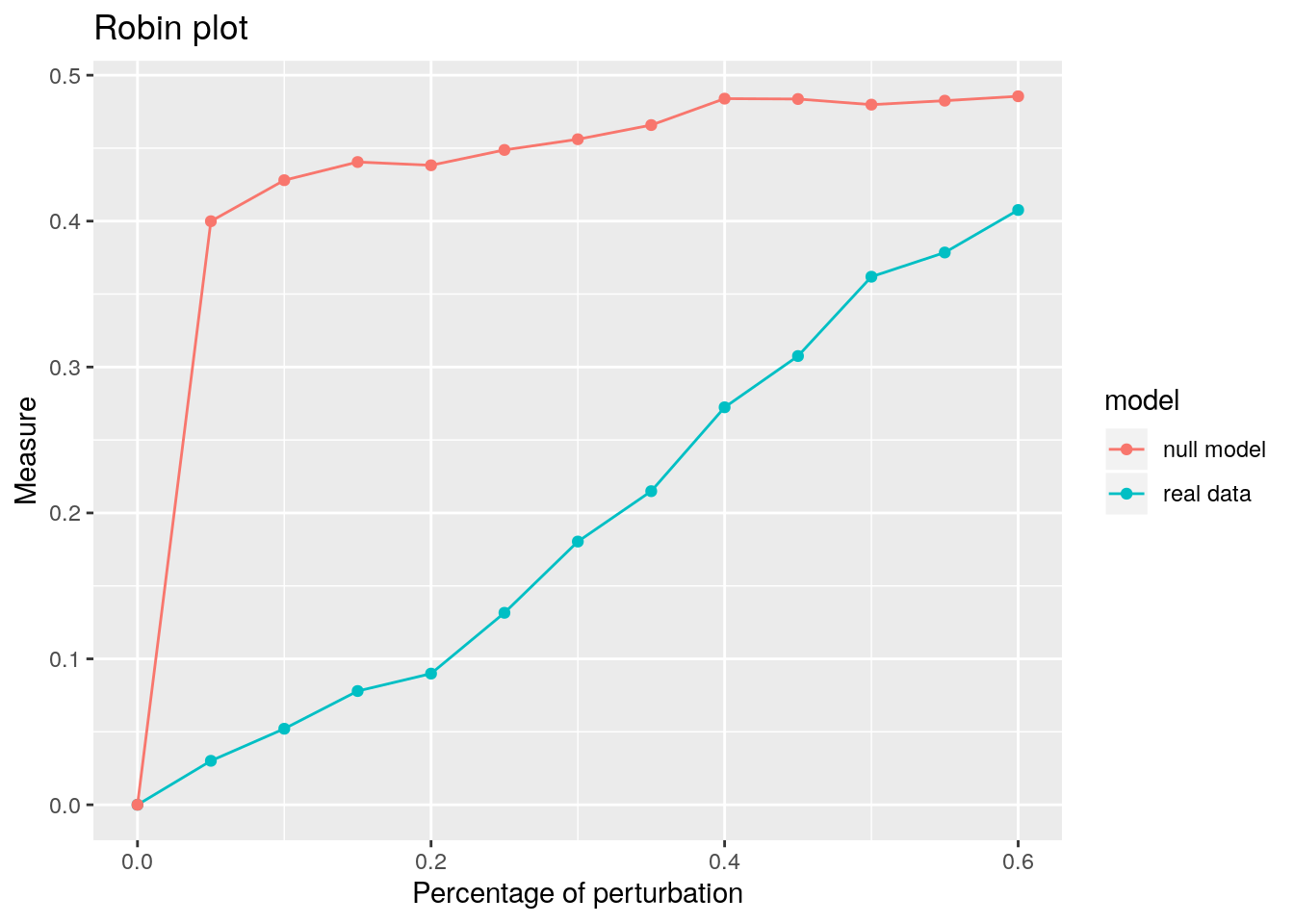# Statistical Tests between Real data and Null model

The differeces between this two curves are tested using: - Functional data analysis - Gaussian Process - Area Under the Curve (AUC)

``````robinFDATest(graph=graph, model1=proc\$Mean, model2=proc\$MeanRandom,
measure="vi")``````
``````##  "First step: basis expansion"
## Swapping 'y' and 'argvals', because 'y' is  simpler,
##   and 'argvals' should be;  now  dim(argvals) =  13 ;  dim(y) =  13 x 20
##  "Second step: joint univariate tests"
##  "Third step: interval-wise combination and correction"
##  "creating the p-value matrix: end of row 2 out of 9"
##  "creating the p-value matrix: end of row 3 out of 9"
##  "creating the p-value matrix: end of row 4 out of 9"
##  "creating the p-value matrix: end of row 5 out of 9"
##  "creating the p-value matrix: end of row 6 out of 9"
##  "creating the p-value matrix: end of row 7 out of 9"
##  "creating the p-value matrix: end of row 8 out of 9"
##  "creating the p-value matrix: end of row 9 out of 9"
##  "Interval Testing Procedure completed"``````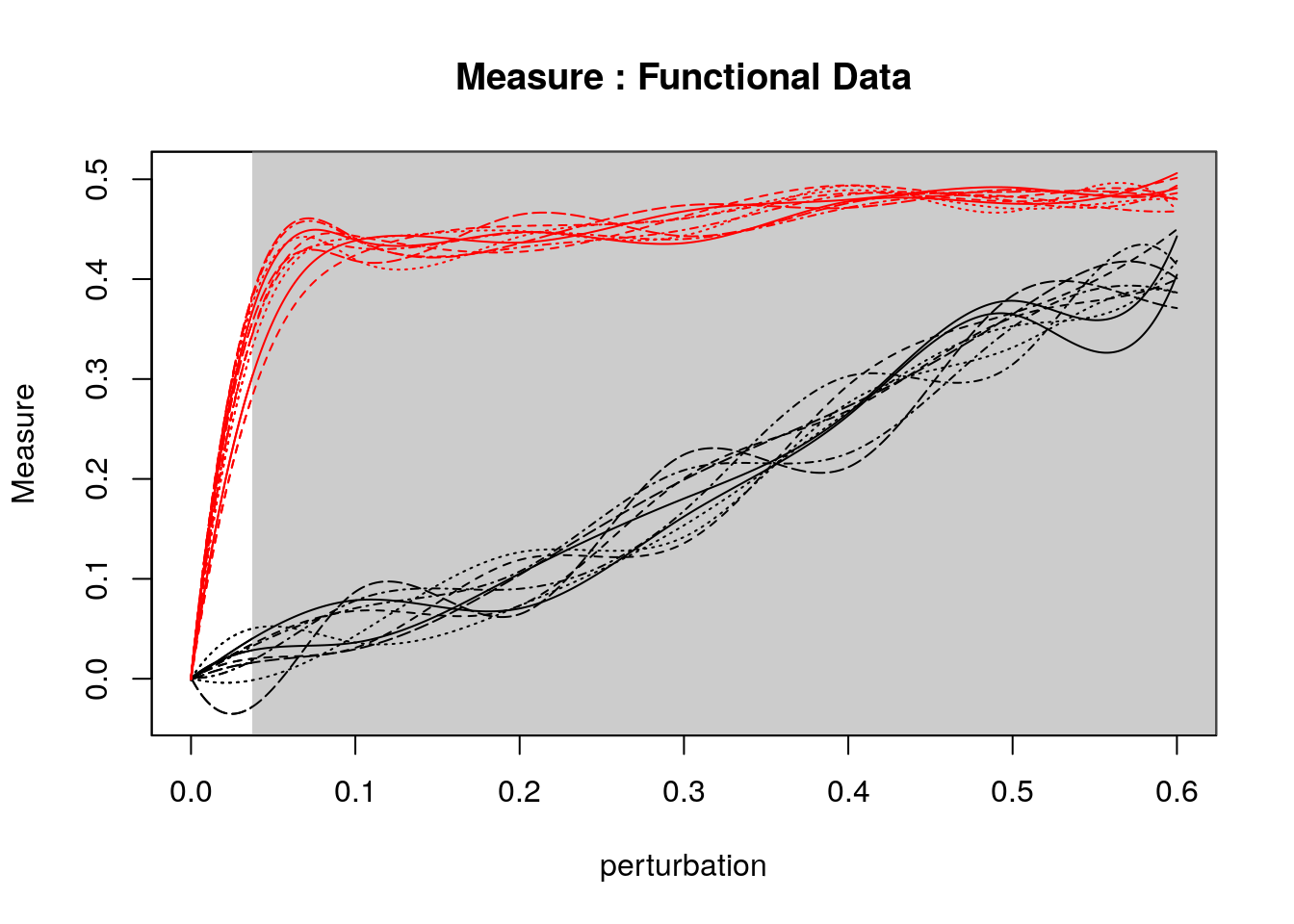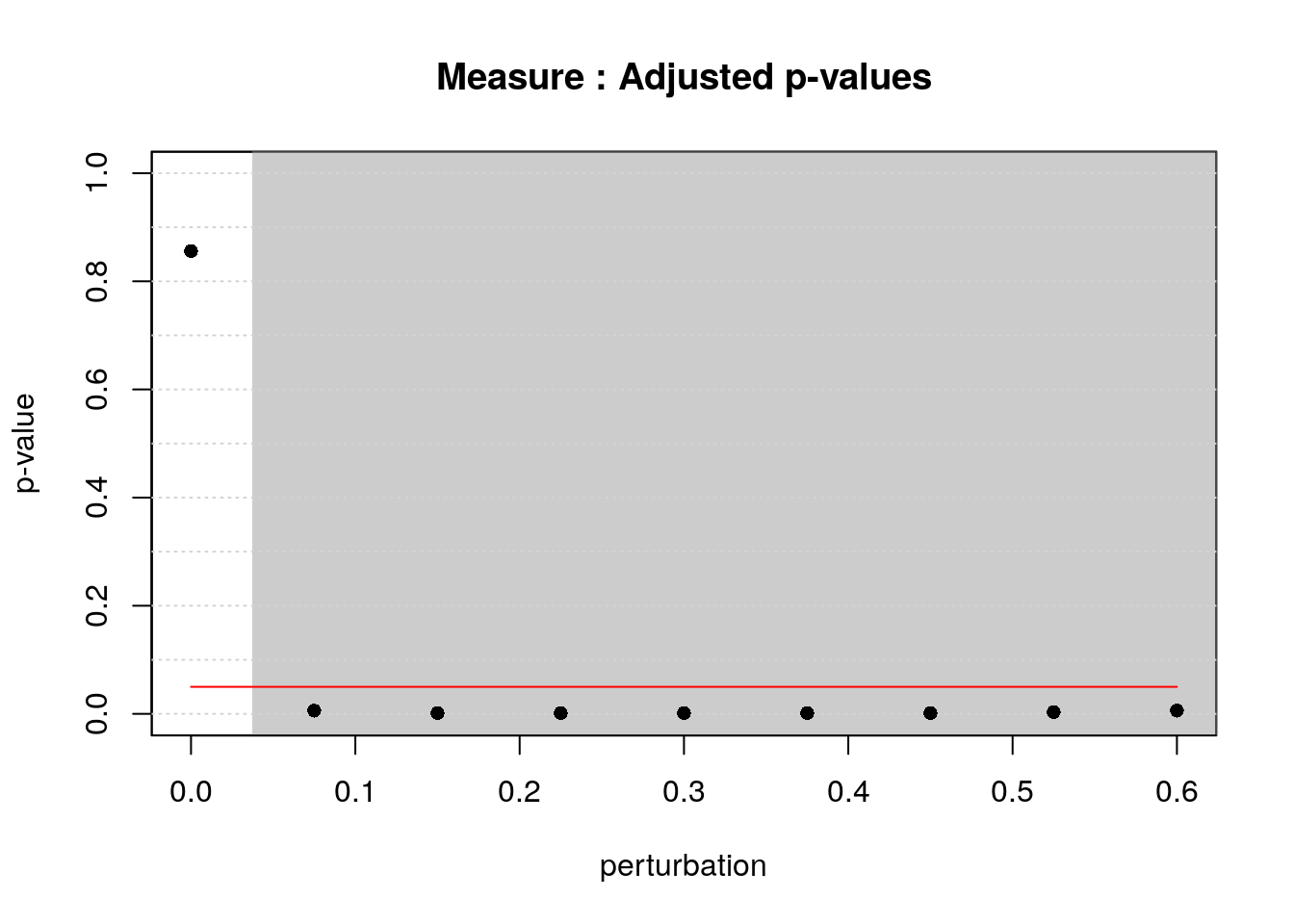``````## \$ask
##  TRUE``````

The first figure represents the stability measure plot on the clustering obtained via louvain algorithm. The second one represents the corresponding adjusted p-values of the Interval Testing procedure. Horizontal red line corresponds to the critical value 0.05.

``robinGPTest(ratio=proc\$ratios)``
``````##  Profile  1
##  Profile  2``````
``##  60.36058``

The output is the Bayes Factor

``````robinAUC(graph=graph, model1=proc\$Mean, model2=proc\$MeanRandom,
measure="vi")``````
``````## \$area1
##  0.1150428
##
## \$area2
##  0.2645781``````

The outputs are the area under the two curves

# Comparison Two different Methods

To choose among different community detection algorithms the one that better fits the network of interest, the fuction robinCompare has to be used.

In this example we take the Fast Greedy and Louvain algorithms.

We firstly plot the communities dectected by both algorithms.

``````membersFast <- membershipCommunities(graph=graph, method="fastGreedy")
membersLouv <- membershipCommunities(graph=graph, method="louvain")
plotComm(graph=graph, members=membersFast)``````
``plotComm(graph=graph, members=membersLouv)``

Secondly, we compare the with robinCompare function.

``````comp <- robinCompare(graph=graph, method1="fastGreedy",
method2="louvain", measure="vi", type="independent")``````
``````##  31
##  61
##  92
##  123
##  153
##  184
##  215
##  245
##  276
##  306
##  337
##  368``````

Thirdly, we plot the two curves of the two compared methods.

``````plotRobin(graph=graph, model1=comp\$Mean1, model2=comp\$Mean2, measure="vi",
legend=c("fastGreedy", "louvain"), title="FastGreedy vs Louvain")``````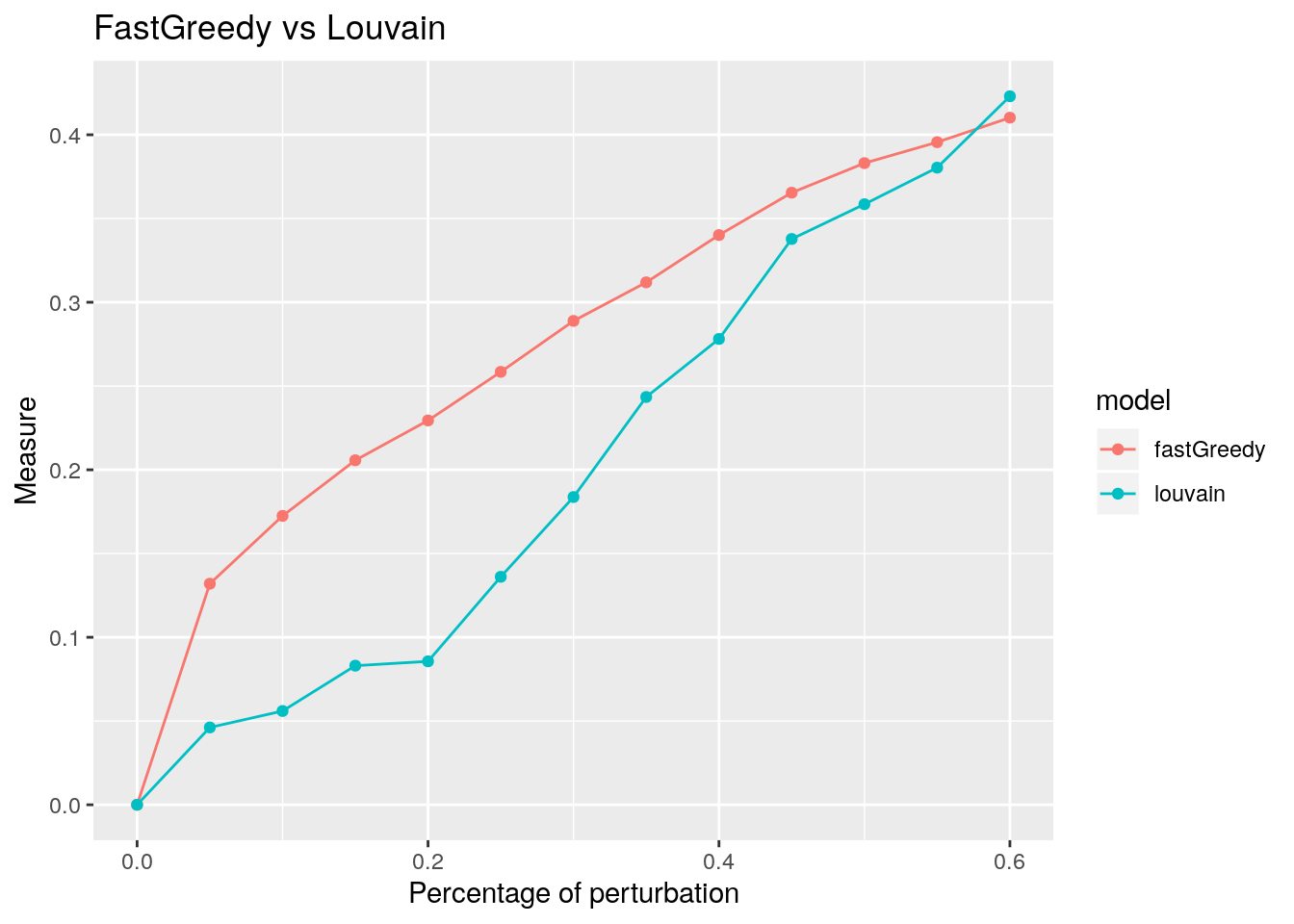In this example, the Louvain algorithm fits better the network of interest, as the curve of the stability measure assumes lower values than the one obtained by the Fast greedy method.

# Statistical Tests between two community detection algorithms

The following procedures test the statistical differeces between the two curves using two different methods

``robinFDATest(graph=graph, model1=comp\$Mean1, model2=comp\$Mean2, measure="vi")``
``````##  "First step: basis expansion"
## Swapping 'y' and 'argvals', because 'y' is  simpler,
##   and 'argvals' should be;  now  dim(argvals) =  13 ;  dim(y) =  13 x 20
##  "Second step: joint univariate tests"
##  "Third step: interval-wise combination and correction"
##  "creating the p-value matrix: end of row 2 out of 9"
##  "creating the p-value matrix: end of row 3 out of 9"
##  "creating the p-value matrix: end of row 4 out of 9"
##  "creating the p-value matrix: end of row 5 out of 9"
##  "creating the p-value matrix: end of row 6 out of 9"
##  "creating the p-value matrix: end of row 7 out of 9"
##  "creating the p-value matrix: end of row 8 out of 9"
##  "creating the p-value matrix: end of row 9 out of 9"
##  "Interval Testing Procedure completed"``````